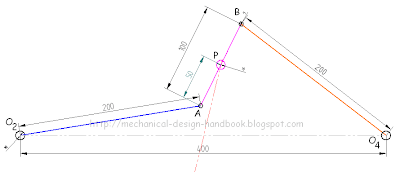## Posts

Showing posts from December, 2010

### Improve math skills of your kids - Learn step-by-step arithmetic from Math games

Math: Unknown - Step-by-step math calculation game for iOS.

Math: Unknown is much more than a math game. It is a step-by-step math calculation game which will teach users how to calculate in the correct order rather than just asking only the final calculated results.

The app consists of four basic arithmetic operations which are addition, subtraction, multiplication and division. In order to get started, users who are new to arithmetic can learn from animated calculation guides showing step-by-step procedures of solving each type of operation. It is also helpful for experienced users as a quick reference.

Generally, addition and subtraction may be difficult for users who just start learning math especially when questions require carrying or borrowing (also called regrouping). The app helps users to visualize the process of carrying and borrowing in the way it will be done on paper. Once users understand how these operations work, they are ready to learn multiplication and division.

For most students, division is considered as the most difficult arithmetic operation to solve. It is a common area of struggle since it requires prior knowledge of both multiplication and subtraction. To help users understand division, the app uses long division to teach all calculation procedures. Relevant multiplication table will be shown beside the question. Users will have to pick a number from the table which go into the dividend. Multiplication of selected number and divisor is automatically calculated, but the users have to do subtraction and drop down the next digit themselves. Learning whole calculation processes will make them master it in no time.

Math: Unknown is a helpful app for students who seriously want to improve arithmetic calculation skills.

### Chebyschev Straight-line MechanismThe Chebyschev linkage is a mechanical linkage that converts rotational motion to approximate straight-line motion.

It was invented by the 19th century mathematician Pafnuty Chebyschev who studied theoretical problems in kinematic mechanisms. One of the problems was the construction of a linkage that converts a rotary motion into an approximate straight line motion. This was also studied by James Watt in his improvements to the steam engine. (Read more info about Watt Straight-line Mechanism)
The straight-line linkage of Chebyschev confines the point P — the midpoint on the link AB — on a straight line at the two extremes and at the center of travel. Between those points, point P deviates slightly from a perfect straight line. The proportions between the links are

O2O4 : O2A : AB = 200 : 250 : 100 = 4 : 5 : 2

Point P is in the middle of AB. This relationship assures that the link AB lies vertically when it is at the extremes of its travel.

Source: http://en.wikipedia.org/wiki/Chebyshev…

### Watt Straight-Line MechanismWatt's linkage (also known as the parallel linkage) is a type of mechanical linkage invented by James Watt to constrain the movement of a steam engine piston in a straight line. The idea of its genesis using links is contained in a letter he wrote to Matthew Boulton in June 1784.
"I have got a glimpse of a method of causing a piston rod to move up and down perpendicularly by only fixing it to a piece of iron upon the beam, without chains or perpendicular guides [...] and one of the most ingenious simple pieces of mechanics I have invented." This linkage does not generate a true straight line motion, and indeed Watt did not claim it did so.

Watt's straight-line mechanism is used in the rear axle of some car suspensions. It intends to prevent relative sideways motion between the axle and body of the car. Watt’s linkage approximates a vertical straight line motion more closely, and does so while locating the center of the axle rather than toward one side of the vehicle.

I…